# AP Board 8th Class Maths Solutions Chapter 10 Direct and Inverse Proportions Ex 10.2

AP State Syllabus AP Board 8th Class Maths Solutions Chapter 10 Direct and Inverse Proportions Ex 10.2 Textbook Questions and Answers.

## AP State Syllabus 8th Class Maths Solutions 10th Lesson Direct and Inverse Proportions Exercise 10.2Question 1.
Observe the following tables and fmd which pair of variables (x and y) are in inverse proportion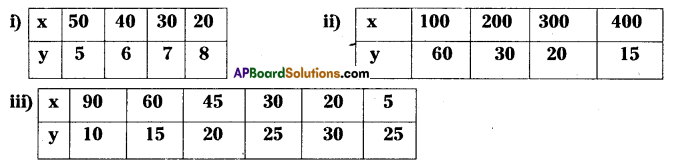Solution:
i) From the given table if the value of x is decreases then the value of ‘y’ is increases.
∴ x, y are in inverse proportion.
ii) From the given table if the value of x is increases then the value of y is decreases.
∴ x, y are in inverse proportion.
iii) From the given table the value of x is decreases then the value of y is increases.
∴ x, y are in inverse proportion.Question 2.
A school wants to spend ₹6000 to purchase books. Using this data, fill the following table.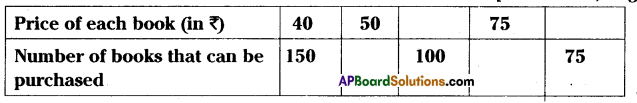Solution: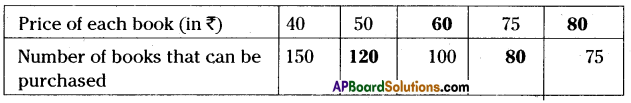Question 3.
Take a squared paper and arrange 48 squares in different number of rows as shown below.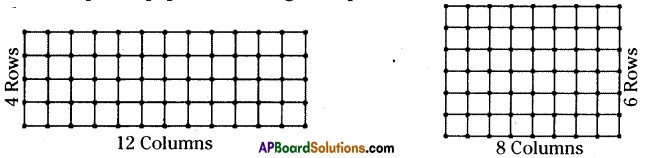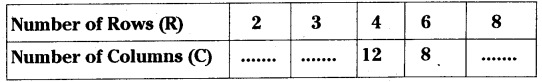What do you observe? As R increases, C decreases
(i) Is R1:R2 = C2:C1?
(ii) Is R3:R4 = C4:C3?
(iii) Is R and C inversely proportional to each other?
(iv) Do this activity with 36 squares.
Solution:
(i) Is R1:R2 = C2:C1
⇒ 2 : 3 = 16 : 24

(ii) Is R3:R4 = C4:C3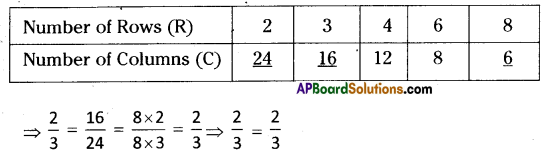R1:R2 = C2:C1

(iii) R3:R4 = C4:C3
⇒ 4 : 6 = 8 : 12
$$\frac{4}{6}=\frac{8}{12}=\frac{4 \times 2}{6 \times 2}=\frac{4}{6} \Rightarrow \frac{4}{6}=\frac{4}{6}$$ =
∴ R3:R4 = C4:C3

(iv) Do this activity with 36 squares.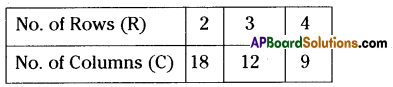From the above table we can conclude that if number of rows are increases then number of columns are decreases.